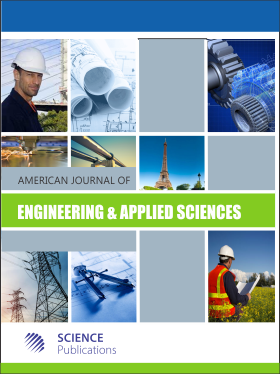Frequency: Quarterly
ISSN: 1941-7020 (Print)
ISSN: 1941-7039 (Online)
Research Article Open Access

# Machine Motion Equations at the Internal Combustion Heat Engines

Florian Ion T. Petrescu1
• 1 Bucharest Polytechnic University, Romania
American Journal of Engineering and Applied Sciences
Volume 8 No. 1, 2015, 127-137

DOI:

Published On: 21 April 2015

How to Cite: Petrescu, F. I. T. (2015). Machine Motion Equations at the Internal Combustion Heat Engines. American Journal of Engineering and Applied Sciences, 8(1), 127-137. https://doi.org/10.3844/ajeassp.2015.127.137

## Abstract

This paper presents an algorithm for setting the dynamic parameters of the classic main mechanism of the internal combustion engines. One presents the dynamic, original, machine motion equations. The equation of motion of the machine that generates angular speed of the shaft (which varies with position and rotation speed) is deduced by conservation kinetic energy of the machine. An additional variation of angular speed is added by multiplying by the coefficient dynamic (generated by the forces out of mechanism). Kinetic energy conservation shows angular speed variation (from the main shaft) with inertial masses, while the dynamic coefficient introduces the variation of ω with forces acting in the mechanism. Deriving the first equation of motion of the machine it obtains the second equation of motion dynamics. From the second equation of motion of the machine one determines the angular acceleration of the motor shaft. It shows the distribution of the forces (on the main mechanism of the engine) to the internal combustion heat engines. Dynamic, the velocities can be distributed in the same way as forces. Practically, in the dynamic regimes, the velocities have the same timing as the forces. The method is applied separately for two distinct situations: When the engine is working on a compressor and into the motor system. For the two separate cases, two independent formulas are obtained for the engine dynamic cinematic (forces speeds). Calculations should be made for an engine with a single cylinder.